# Chapter 8: chemical kinetics – reactions in solution

The big difference between a solution and a gas is the presence of a solvent. A viscous solvent means that there are more collisions between the molecules of reactant and the molecules of solvent, slowing down the molecules. The first obligation to obtain a reaction is that the reactants have to be close to each other. In a still solution, the species move by diffusion (if the solution is mixed manually or mechanically, the main transport process is called convection but the diffusion still acts with a minor influence). Once the reactants are nearby, they are caged in the solvent and remains nearby for a given time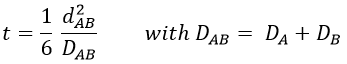with DI the coefficient of diffusion of the species I. The reaction is the result of a vibrational movement. As for reactions of several steps, the global speed of a reaction will depend upon the slowest process. Diffusion can be the limiting factor.

Diffusion controlled reactions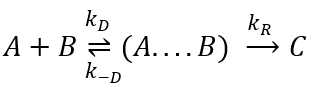There are thus two steps in the reaction. The limiting step is the diffusion if k-D<<kR and the reaction is the limiting step if kR<<k-D. We use the assumption of stationarity for the concentration of the complex, i.e. the products C are formed at the same speed that the reactants are consumed.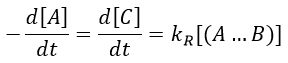The concentration of the intermediate species is unknown but can be determined from the concentrations of the reactants if we know the constants of reaction.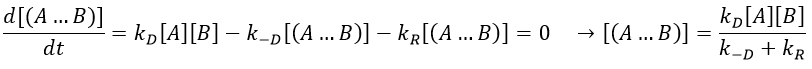We can now put this expression in the speed of reaction: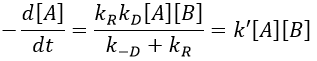There are two limit cases:

• kR>>k-D: k’=kD, the reaction step is negligible in comparison to the time it takes to the reactants to meet. The reaction is limited by the diffusion.
• kR<<k-D: k’=kR.kD/k-D~kR, we can consider that the diffusion to approach or to go away from the other species is similar, so the speed of reaction is limited by the reaction itself.

Flow calculation

At the beginning of the experiment, i.e. t=0, the reactants A and B are uniformly distributed in the solution. No reaction took place yet and the solution is homogeneous. A short time after t=0, some reactants have formed products and a gradient of concentration can exist locally. The flow of diffusion J is given by the law of Fick: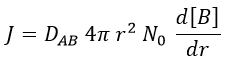There is a flow of B towards A which is in the middle of a sphere or radius r. We integrate this expression from the smallest distance (dAB, i.e. the sum of the radii of A and B) to infinity.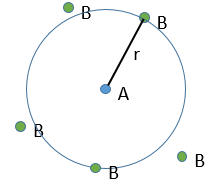at r=dAB, [B]=0: the reaction is limited by the diffusion so we can consider that molecules of B that enter in contact with A react immediately. At an infinite distance r= ∞, the concentration of B is not affected by the presence of A and [B]=[B]0, the initial homogeneous concentration of B in the solution. Noting CB(r) the local concentration of B, the integration gives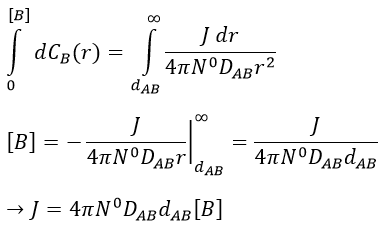This value is the flow of molecules of B moving towards one molecule of A by unit of time. There are obviously more than one molecule of A in the solution.

At the stationary state,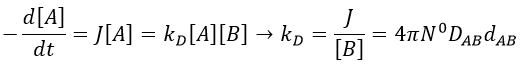For instance, if we consider two usual molecules, dAB=0.6nm and DAB=2. 10-9 m2s-1, then kD~9.109mol-1ls-1. If the constant of reaction is of this order, then the reaction is limited by the diffusion. The constant is 20-100 times smaller in gaseous phases.

The equation of Stokes-Einstein connect the diffusion of a species with the viscosity of the solvent.We can inject this expression in kD if A and B have similar dimensions to find that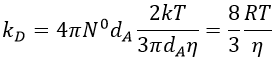In viscous solvents, if kD has this order of value, then the reaction is limited by the diffusion. A correction has to be considered if the species are charged: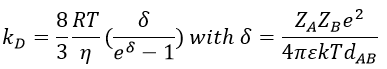If the charges of A and B are opposite, then there is an attraction between the species and kD+->kD. If the charges are of same sign, then there is a repulsion and kD++<kD.

Speed of complex chemical reactions

The theories we have seen before are valid for elementary reactions only and approximations have to be done to study more complex reactions.

Parallel reactions

In this case several reactions of the same order (m=1 or 2) involve the same reactant.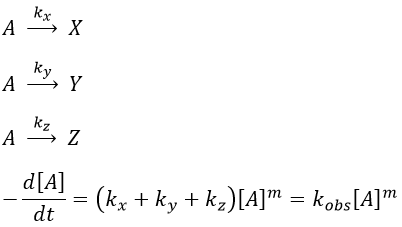The experimental constant kobs is easily determined.  The ratio of the concentrations of the products is equal to the ratio of the constants of reaction.

If there are two reactants, we put one of them in excess to obtain a pseudo order 1 reaction to determine the constant of reaction.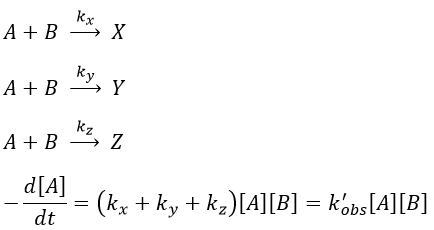if [B]>>>[A] then k’obs[A][B]=k”obs[A]  with k”obs=k’obs[A].

example: chloration of the tertiobutylbenzene

A chlorine atom can be added on tertiobutylbenzene on three different spots.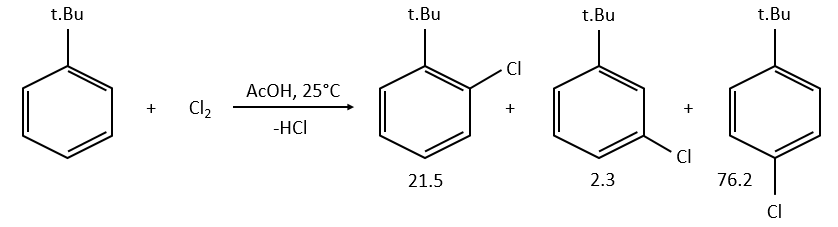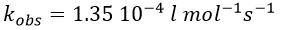Knowing the global constant of reaction and the proportions of the three products, we can determine the constants of reaction of each reaction.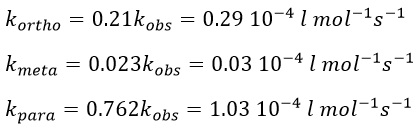Parallel reaction with different orders

• with a single reactant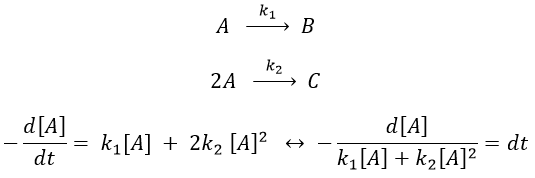Luckily, mathematics give the solution for such an integral: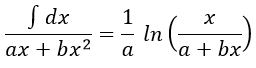As a result,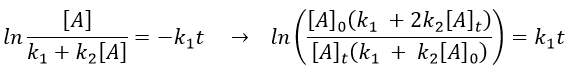The relation is complex and the evolution of the concentration has to be studied through numerical programs. We can estimate the constants of speed with the method of initial speed.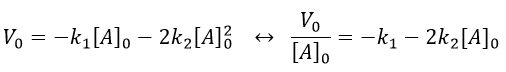If we plot V0/[A]0 vs [A]0, we find an approximated value of k1 at the intersection of the ordinate with the curve and of k2 from the slope of the curve.

example of reaction: behaviour of the cis-trans- cycloocta 1,5-diene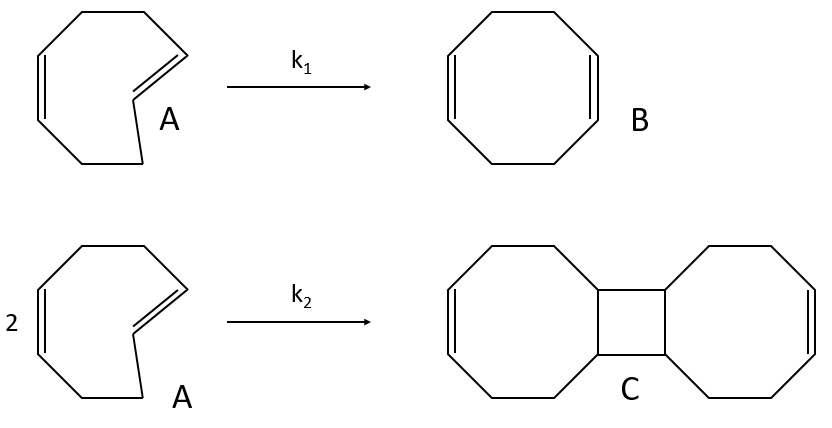A difference with the case of parallel reactions of same order is that the ratio of the concentration of the product is not equal to the ratio of the constants of speed. If we measure the formation of the products, we find that the product B is produced faster and faster with regards to the product C: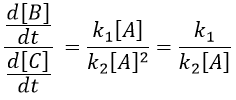Note that even if k2>k1, it means that the formation of B can be faster than the formation of C, immediately or at a given point of the reaction, as soon as the concentration of A is small enough. For instance, given an experiment with a concentration of A of 10-4mol/l, then k2 has to be 10000 times larger than k1 to obtain approximatively the same speed of formation for both products. As the reactions goes on, the speed of formation of C decreases furthermore.

Reaction involving a second reactant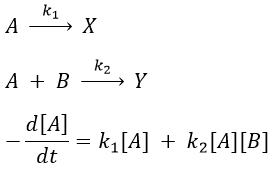We can simplify the problem with an excess of B so the second reaction becomes a pseudo-order 1 reaction.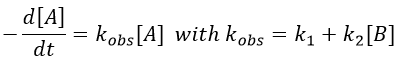Reversible reactions of order 1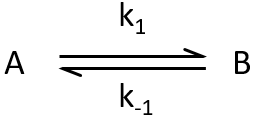The constant of equilibrium K is the ratio of the concentration at the equilibrium of the products over the one of the reactants.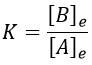There is no notion of kinetics in this expression of the constant of equilibrium. But if we perturb the equilibrium, for instance by an abrupt modification of the temperature, the system has to adapt itself to the new conditions and the concentrations of the species varies.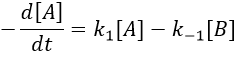We can define x the deviation to the concentration of equilibrium (noted with a subscript e) such as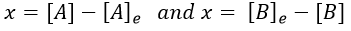The evolution of x is independent of the current concentration and is given by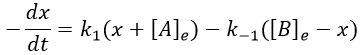When the system reach its new equilibrium, the concentrations and x do not vary anymore.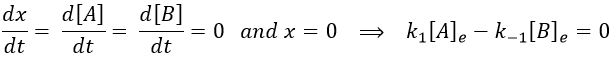As a result, we obtain a new formulation of the constant of equilibrium of the reaction: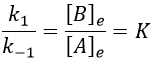For a small perturbation of the equilibrium,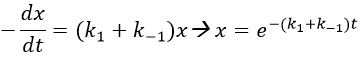The transition between two equilibrium states is thus given by an exponential. If we plot the ln x versus the time, the slope of the curve gives the sum of the constants of speed k1+k-1. The characteristic time of relaxation tau to pass from one state of equilibrium at a temperature T to another one at T’ is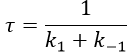Knowing K and τ, we can find k1 and k-1.

Example: racemisation of two optically active enantiomers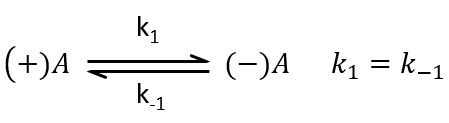If we start from a solution with only one of the two enantiomers, there will be a spontaneous racemisation of the species in solution.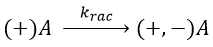We can measure the variation of the angle of polarisation (optical activity α) of the solution over time.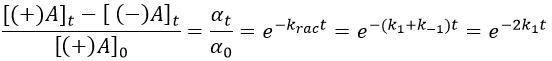α tends towards zero because the optical activities of the enantiomers cancel each other. We see an activity when there is a overpopulation of one of the species.

Consecutive reactions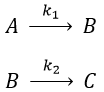The concentrations of the three species can be followed.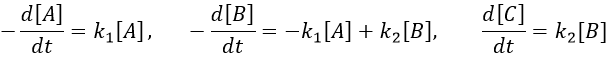B is formed by the first reaction and is consumed by the second one, there is thus two components to the evolution of its concentration, one being positive and one being negative.

The first reaction is an order 1 reaction and the concentration of A is given by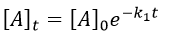This expression can be injected in the expression for B.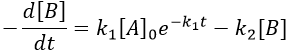It is a differential equation the solution of which is obtained by the sum of a general solution and of a particular expression. The solution is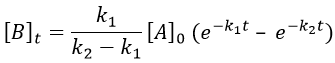As the ratios between reactants and products is 1:1, the sum of the concentrations of the three species is equal to the concentration of A we put initially.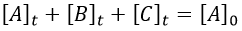At any time, the concentration of the final product C is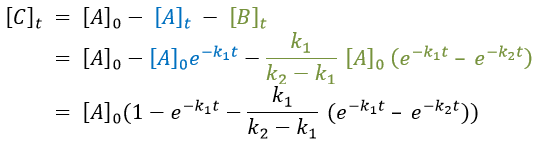After an infinite time, A and B are not in solution anymore and only the product C remain in solution.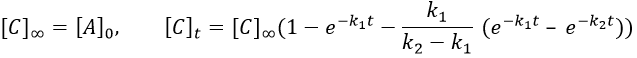The concentration of the intermediate product is highly dependent of the speeds of both reactions and shows a maximum at t=tBmax.

At t<tBmax, there is still plenty of reactant A that can form B. The concentration of B is not large enough to form quickly the final product C of the reaction. As a result, there is an accumulation of B. After tBmax, the rate of formation of B is smaller than its consumption.

If k2>>k1, then B is very reactive and does not accumulate. We can estimate that [B]t»0. If k2<<k1, then B is an intermediate with a long life time and that can accumulate up to a given concentration.

The concentration of C remains low during a period of induction and the evolution of C is not given by a simple function. We cannot obtain a simple kobs from its measurement. k1 can be measured from the concentration of A and k2 is found numerically from the expression of C or of tBmax.

Approximation of the stationary state

The previous case was still quite simple and involved only reactions of order 1. In more complex cases, we use the theorem of the stationary state. The hypothesis is that the concentration of the intermediate product is constant, i.e. it is consumed as fast as it is formed.

In the previous case, we would have obtained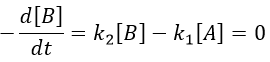It gives us the concentration of B as a function of [A]: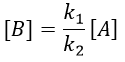The expression for the concentration of A is known so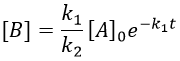This expression, curiously dependant of the time for a species the concentration of which is considered as constant, can be inserted in the expression of C.We can integrate this expression to obtain an expression of the concentration of C as a function of the time.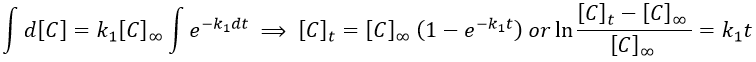A is thus consumed in k1 and C is formed in k1 too. It is coherent with the fact that B disappears as soon as it is formed.

Reversible reaction of order 1 followed by a reaction of order 2

This case seems harder to solve.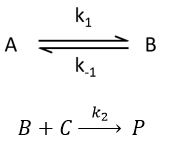Depending on the constants of speeds, several cases can be sorted:

• the equilibrium and the consumption of B have approximatively the same speed,
• the equilibrium is slow and B is consumed quickly,
• the equilibrium is quickly made with regards to the speed of the second reaction.

In a general way,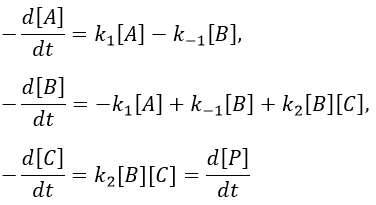We make the assumption of a stationary state for B.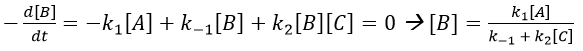We insert this expression in the expression of A.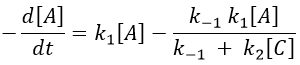We still have two different concentrations that varies with the time in this expression ([A] and [C]). To obtain a solution, we need to find some limit conditions.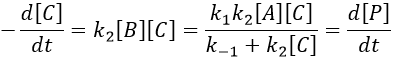The final concentration of product P is either limited by the initial concentration of A or by the one of C, depending on which one is the smallest and which one is in excess. If we put C in excess, then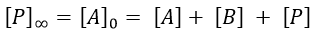If [B] << [A]+[P], i.e. the case for which the hypothesis of stationarity is valid, then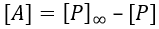and the expression can be simplified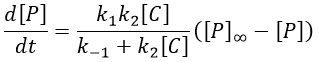If C was put in large excess, then we can consider its concentration as a constant and we find a pseudo order 1 reaction (k’2=k2[C]).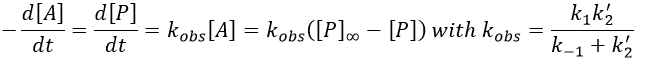We can find the value of k1 if we use several different concentrations of C.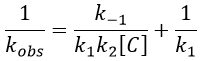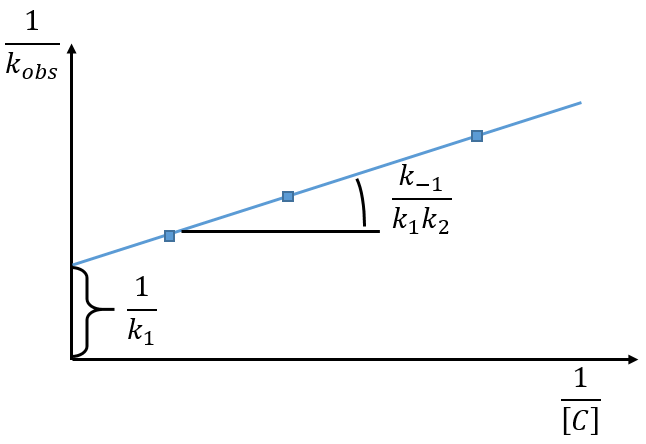The intersection of the axis with the curve gives 1/k1 and the slope of the curve gives k-1/k1k2. However we don’t have the values of k-1 and k2.

There are two limit cases:

1) k2[C]>>k-1

B doesn’t turn back into A. The second step of the reaction doesn’t allow the first reaction to return to the equilibrium. As a result, both reactions can be considered as irreversible, what results in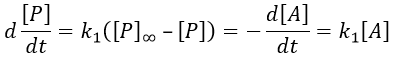In this case, the order of the reaction is 1 even if C is not put in a large excess. [C] can be mathematically removed from the equation. The experimental constant of speed is equal to k1. The first step is thus the limiting step of the reaction.

Example: acetone + Cl2 in an acid middle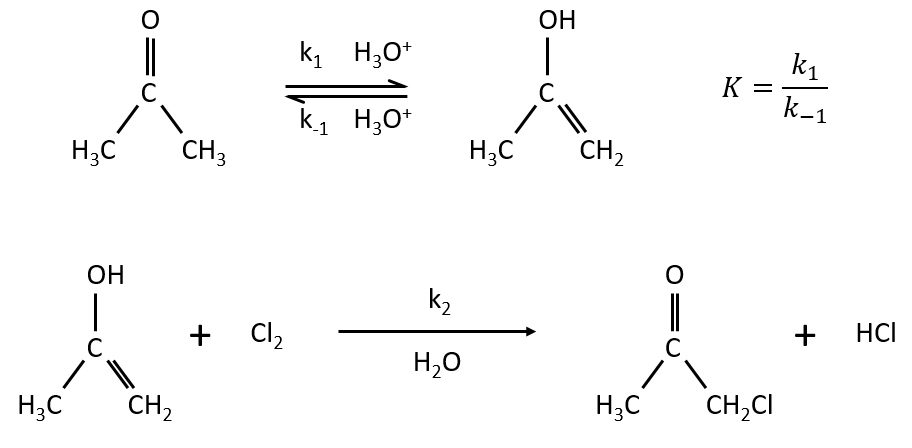2) k2[C]<<k-1

The equilibrium of the first step of the reaction has the time to be reached. It leads to a global reaction of order 2.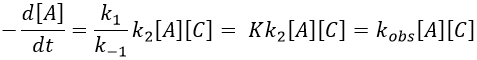If C is in large excess, the reaction is of pseudo-order 1 as seen in the case 1, but if we put a very small concentration of Cl2 instead, then the expression for the speed changes: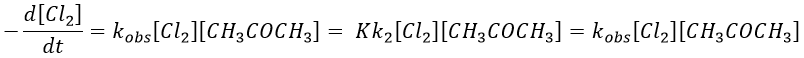Just by a modification of the experimental conditions, we can determine all of the constants of equilibrium of the enol-ketone tautomerism.

Reversible reaction of order 2 followed by an irreversible reaction of order 1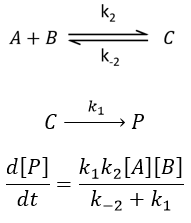if k1>>k-2, then the first reaction has no time to return to the equilibrium and we can assume that the reactions are irreversible. The speed of the global reaction is limited by the first step of the reaction.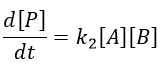In the opposite case (k1<<k-2), we are still in the conditions of an apparent order 2 reaction but the constant of speed involves both reactions

Application: saponification of an ester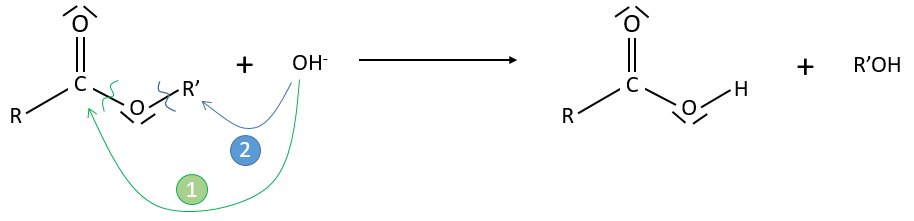Experimental data tell that this reaction is of order 2 with the speed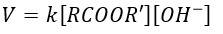However this expression still corresponds to two possible mechanisms. To determine which one is correct, we use an isotope of the oxygen (O 18) in the carbonyl group. The presence of the marked oxygen in the molecule indicates the good mechanism: during the reaction, this oxygen is equivalent to the one of the OH group if the attack takes place on the carbonyl. Some marked oxygens can thus leave the molecule or change of position.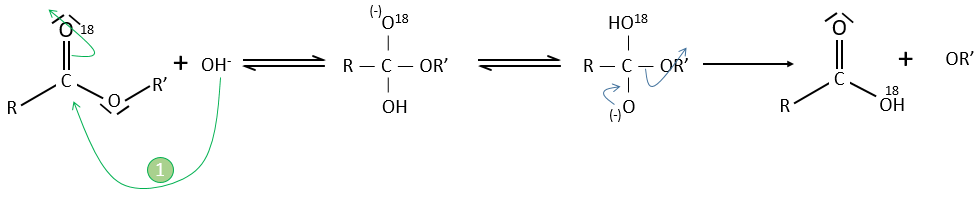If R’O is a good leaving group or not, we have two cases.

• Good leaving group
• Fast elimination, faster than the equilibrium
• The addition determines the speed
• V=k2[RCOOR’][OH]